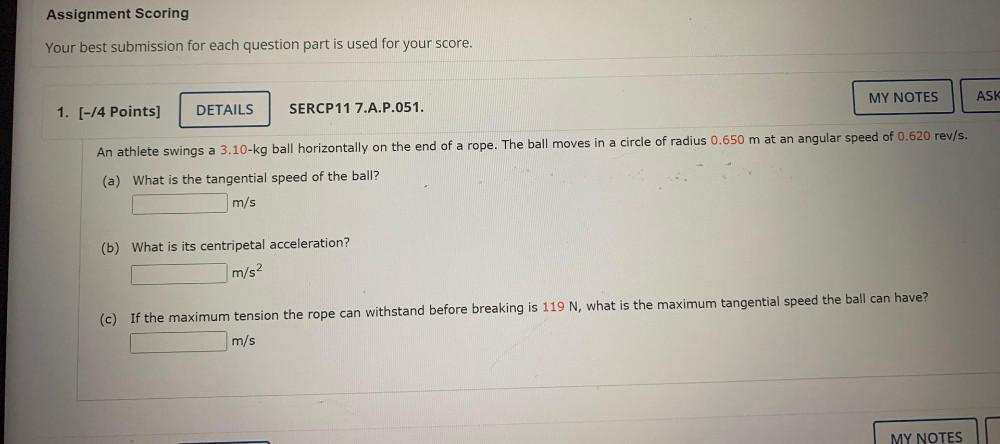Question:

# An athlete swings a 3.10-kg ball horizontally on the end of a rope. The ball moves in a circle of radius 0.650 m at an angular speed of 0.620 rev/s. (a) What is the tangential speed of the ball? (b) WAn athlete swings a 3.10-kg ball horizontally on the end of a rope. The ball moves in a circle of radius 0.650 m at an angular speed of 0.620 rev/s. (a) What is the tangential speed of the ball? (b) What is its centripetal acceleration? (c) If the maximum tension the rope can withstand before breaking is 119 N, what is the maximum tangential speed the ball can have?